StatLect

Sample variance

Given a number of observations, their sample variance measures how far they are spread apart. It is also an estimator of the variance of the population from which the observations have been drawn.Computation

Givenobservations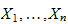having sample mean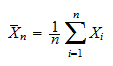there are two main ways to compute the sample variance:

• unadjusted sample variance, also called biased sample variance:• adjusted sample variance, also called unbiased sample variance: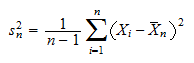When theobservations are independent,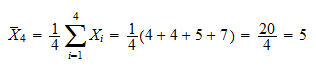is a biased estimator of the population variance, whileis unbiased.

Example

Suppose we observe four realizations:Their sample mean is:Dividing the sum of squared deviations from the mean by the number of observations, we obtain the unadjusted sample variance: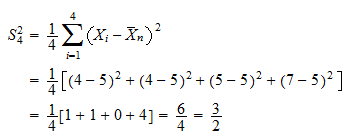Dividing it by the number of observations minus one, we instead obtain the unbiased estimate:More details

The lecture entitled Variance estimation provides more details about the sample variance, including derivations of its properties, examples and exercises.